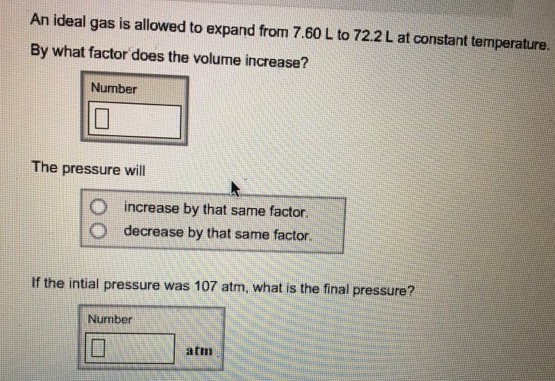Chemistry Practice Problems Chemistry Gas Laws Practice Problems Solution: An ideal gas is allowed to expand from 7.60 L to 7...

🤓 Based on our data, we think this question is relevant for Professor King's class at LONESTAR.

# Solution: An ideal gas is allowed to expand from 7.60 L to 72.2 L at constant temperature. By what factor does the volume increase? The pressure will increase by that same factor. decrease by that same factor. If the initial pressure was 107 atm, what is the final pressure?

###### Problem

An ideal gas is allowed to expand from 7.60 L to 72.2 L at constant temperature. By what factor does the volume increase? The pressure will increase by that same factor. decrease by that same factor. If the initial pressure was 107 atm, what is the final pressure?###### Solution

According to the Boyle's Law, Volume is inversely proportional to PressureView Complete Written Solution

Chemistry Gas Laws

Chemistry Gas Laws

#### Q.  A sample of an ideal gas has a volume of 2.22 L at 279 K and 1.05 atm. Calculate the pressure when the volume is 1.73 L and the temperature is 303 K....

Solved • Sat May 12 2018 14:04:07 GMT-0400 (EDT)

Chemistry Gas Laws

#### Q. What is the volume occupied by 18.6 g of argon gas at a pressure of 1.19 atm and a temperature of 394 K? Express your answer with the appropriate unit...

Solved • Tue Apr 24 2018 10:49:07 GMT-0400 (EDT)

Chemistry Gas Laws

#### Q. A 12 liter tank contains helium gas pressurized to 160 atm. What size tank would be needed to contain the same amount of helium at atmospheric pressur...

Solved • Fri Mar 30 2018 15:36:16 GMT-0400 (EDT)

Chemistry Gas Laws

#### Q. A 12 Liter tank contains helium gas pressurized to 160 atm.How many 3-liter balloons could the 12 Liter helium tank fill?(Keep in mind that an "exhaus...

Solved • Tue Mar 27 2018 11:49:05 GMT-0400 (EDT)